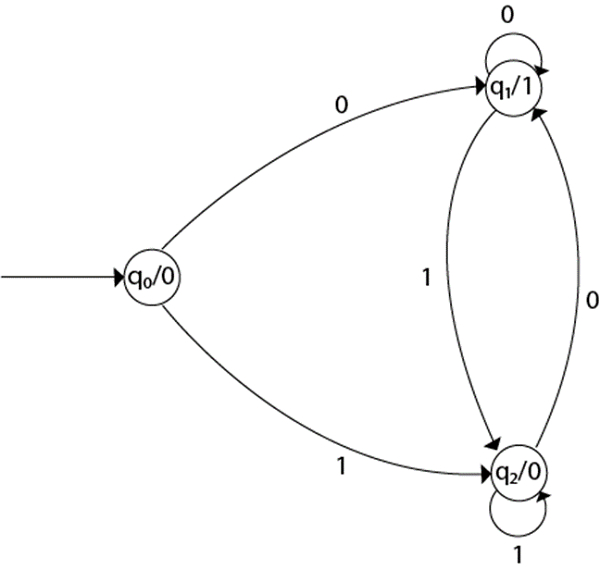# Design a Moore machine to generate 1's complement of a binary number.

Moore machine has 6 tuples, which are as follows −

(Q, q0, Σ, O, δ, λ)

Where,

• Q: Finite set of states
• q0: Initial state of machine
• Σ: Finite set of input symbols
• O: Output alphabet
• δ: Transition function where Q × Σ → Q
• λ: Output function where Q → O

The transition diagram is as follows −## Explanation

• Step 1 − q0 is the start state on input ‘0’ goes to q1 state and on ‘1’ goes to state q2 generating output 0.
• Step 2 − q1 on input ‘0’ goes to q1 itself and on ‘1’ goes to q2 generating output ‘1’.
• Step 3 − q2 on input ‘0’ goes to q1 and on ‘1’ goes to q2 generating output ‘0’.

For instance,

Take one binary number: 1011.

Input

 Input State Output 1 0 1 1 q0 q2 q1 q2 q2 0 0 1 0 0

Let’s construct the transition table for the given language. The table is as follows −

Current StateNext StateOutput
00
->q0q1q20
q1q1q21
q2q1q20